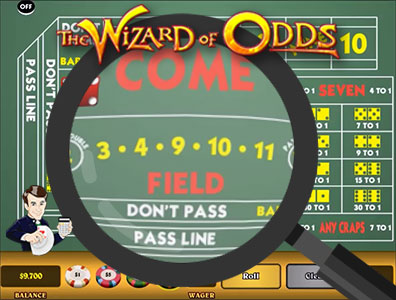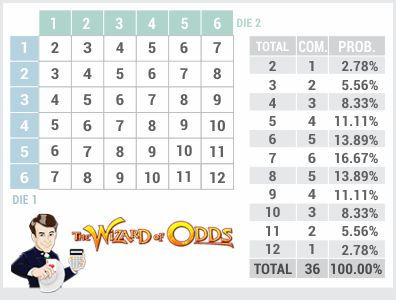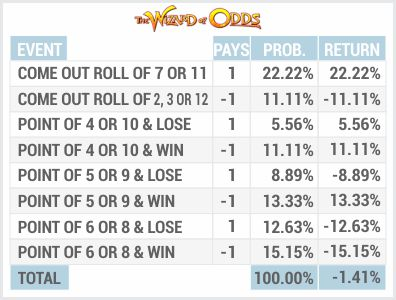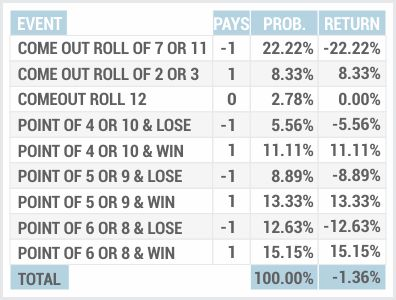## Wizard Recommends

Last Updated: September 18, 2018

# Analyzing the Pass Bet in Craps

Hi, this is Mike and the purpose of this video will be to answer the questions:

• How do I make the pass line bet in craps?
• How do I win it?
• What is the probability of winning?
• And what is the house advantage?First, let's go over the rules of the pass line bet

It's very logical and I love to use flowcharts to explain logical things. You use two ordinary six-sided dice and you roll them. That first roll is called a come out roll but we'll call it the initial roll to keep it in plain simple English. If that initial roll is a 7 or an 11 then the player wins.

If it's not...

...if that initial roll is a 2, 3 or 12 then the pass line bet loses. Otherwise, if it is a 4, 5 or 6, 8, 9 or 10 then the shooter will roll the dice again with the object being to roll whatever that initial roll was. If the new roll is equal to the total of the initial roll, for example, let's say the initial roll was a total of 10 and the second roll is also a 10 then, the pass line bet wins.

If it's not and the new roll is a seven then, the pass line bet loses. That's called a seven-out. It's why seven is generally a bad number in craps. Otherwise, if it's not the initial roll and it's not a seven then, the shooter rolls the dice again, with the same object of trying to roll whatever that initial roll was.

If the next roll equals the initial roll, the pass line bet wins. If it's a seven it loses, otherwise, you roll again and you keep rolling over and over and over again until the new roll either is the initial roll or a seven.

In theory

The bet could go on forever with an infinite number of rolls but it never does. It always resolves eventually and the average number of rolls needed to resolve a pass line bet is 8.5255.

Let's go through an example, shall we?

This is the craps game on my website, Wizard of Odds. I think it's a much-loved application on my site. It shows all the bets in craps, so don't get intimidated by this. We're just going to worry about this pass line bet here. You can tell it's a come out roll because there's a lammer up here that says "OFF"

That means that it's safe to make the pass line bet. Let's bet \$100 on it and roll the dice. The initial roll was a seven so, we won even money.

Let's do another roll

Now the come out roll, this time it was an 11. Again, if the come out roll is a 7 or 11, you win. So let's make a third one, another come out roll, a two. As a reminder, if the come out roll is a 2, 3 or 12, you lose so I lost that one.Let's make another one, a three

Another pass line bet loss because it's a 2, 3 or 12. Let's make another one. Six, okay. Finally, we have a point. The point is a six. That lammer at the top now says "ON" and it's by the number six to remind us, the point is a six, the object is to roll a six and, as always except on the initial roll, you want to stay away from a seven.

So let's roll the dice again, trying to roll a total of six. It's a 10, nothing happens. It's a nine, nothing happens again. The only rolls where something happens at this point is on a six or a seven.

An eight, nothing happens

Four, nine, six, we win. We rolled a six before the seven. We won even money, \$100. Now, it's a new come out roll. Let's go through a few more examples, shall we? It's a new come out roll, let's make our bet on the pass line, and a seven.

Again

If the initial roll is a 7 or 11, you win. I won so let's make a new pass line bet. A four, the point is a four so we want to roll another four. A nine, nothing happens. Six, nothing happens. A seven, seven-out, we lost. Let's make a new pass line bet.

Eight

The point is an eight, so we're trying to roll another eight. Here we go, it's a three, nothing happens, eleven, nothing happens, eight, we won. I think we've covered all the ways the pass line bet can win or lose.

To calculate the odds in craps it's essential that you be able to calculate the probability of any given total with two dice. If you already know that, you can skip this part.Let's label the dice:

Die 1 and Die 2, each one of them can be 1, 2, 3, 4, 5 or 6. In the body of this table here, let's put the total of the two dice. Here you can see every possible total from 2 to 12. You can see that some totals happen more often than others and let's take a closer look at that. The possible totals can be anything from 2 to 12.

Let's count the number of ways each total can happen

Let's take the total to make sure we did it right, good. 6 x 6 is 36, so 36 possible outcomes. This table shows us there's one way to roll a two, two ways to roll a three, three ways to roll a four and so on. To put that in terms of a probability here's the probability of each total.

Let's put that in a percentage basis

You can see the most likely total here is the dreaded seven in craps with a probability of 16.67% or 6/36. The probability of five, for example, is 5/36 or 13.89%. Hopefully, you understood that. Let's go on to the next part here of calculating the expected return of the pass line bet.

As you know...

...there's a host of possible outcomes of the bet besides just winning and losing, there's different ways of winning and losing. One of them is a come out roll of 7 or 11, that pays even money, so represent that by a pays of one. As we saw from the previous part, the probability of rolling a 7 is 16.67% and 11 is 5.56% so, the probability of winning on the come out roll is 22.22%.

Another possible outcome is that the come out roll is a 2, 3 or 12. That happens, the player loses, so we can represent the win there as -1, meaning the player lost his initial bet. So as we saw from the previous part, the probability of rolling a 2 is 2.78%, a 3 is 5.56% and 12 is another 2.78%. The probability of losing on the come out roll is 11.11%.

Another possible outcome is that the point is a 4 or a 10, and then the player goes on to win. We saw from the previous part, the probability of rolling a 4 is 8.33%, and a 10 is also 8.33%. The probability of rolling a 4 or 10 on the come out roll is 16.67%, but you don't always win. You only win one-third of the time when the point is a 4 or a 10.

Why is it one in three?

Well, let's consider a point of a four, for example. There's three ways to roll a four and there are six ways to roll a seven. Given that a roll was a four or a seven, how shall I put this? There are three ways it could be a four, and nine total ways it can be four or seven, so three divided by nine reduces to one divided by three.

The probability of rolling a 4 or a 10 in the come out roll and then subsequently winning is 5.56%. Another possible outcome is rolling a point of four or ten and then losing.

That's a similar formula except...

...the probability of rolling the 4 or 10 is still 8.33%, but the probability of losing it, is six out of nine or two divided by 3 because there's six ways to lose and nine ways to win or lose. Six divided by nine reduces to two divided by three, so the probability of rolling a 4 or a 10 on the come out roll and then subsequently losing is 11.11%.

Another possible outcome is rolling a five or nine on the come out roll and then subsequently winning.

As we saw in the previous part, the probability of rolling a five or a nine is 22.22 %, 11.11% each. Given that you've rolled a 5 or a 9, the probability of going on to win is 4 divided by 10. Why is it 4 divided by 10? Let's consider a point of five for example.

There's four ways to roll a five, and six ways to roll a seven. There's 4 plus 6 or 10 ways to roll a 4, or I mean 5 or a 7 and 4 of those 10 ways result in a win. Given that the point is a 4 or a 10, the probability of going on to win is 40%.

The overall probability of rolling a come out roll a five or nine and going on to win is 8.89%. Likewise, probability of the come out roll of a five or nine and then going on to lose is that of 11.11% of rolling the five or nine to begin with and then 60%, because once you've rolled a five or a nine, there's a 60% chance of losing because there's six ways of losing and ten ways you can win or lose.

That doesn't look right. Yes. There's a 13.33% chance of rolling a five or nine on the come out roll and then going on to lose.

Another possible outcome is rolling a six or eight on the come out roll and then going on to win

The probability of rolling a six or an eight on the come out roll is 27.78%, but again, not all of those ways result in a win. Whether the point be a six or an eight, there are five ways to win and six ways to lose. Given that the point is a 6 or an 8, the chances of winning are 5 and 11. All things considered, the probability of rolling a six or an eight on the come out roll and then going on to win is 12.63%.

Likewise, the probability of rolling a six or an eight on the come out roll and then going on to lose, so the probability of rolling the six or the eight to begin with, is 13.89% plus 13.89%, one for the six, one for the eight, times six ways for the probability of rolling a seven and losing, divided by five ways you can win, and six ways you can lose.

Given that the point is a 6 or an 8, the chances of losing are 6 and 11. Overall, the probability of rolling a six or eight and eight on the come out roll and then going on to lose is 15.15%. Let's add all this up and hope it adds to 100%. Yes, it does.The return is simply...

...the probability of any given event, multiplied by how much the player wins if that event does happen. The win is represented by a -1 if he loses. There's all the possible what I call the contributions to the pass to the return. Let's add all those up and we get -1.41%.

That means that for every dollar the player bet on the pass line, he can expect to lose 1.41 cents or 1.41% of whatever his total bet was. As long as I've come this far, I may as well address the don't pass bet, which is almost the opposite of the pass line bet.

Let's modify all the hard work we already did for our pass line bet table:

Okay, on the don't pass bet, if the come out roll is a 7 or 11, then it loses. If the come out roll is a two or a three, then the don't pass bet wins. The probability of the two is 2.78%, and a three is 5.56%. The probability of winning the don't pass on the come out roll is 8.33%.

The only thing that is not the mirror opposite, is what happens on a come out roll of a 12. That will cause the pass line bet to win, but it causes the don't pass bet to only push. We'll put a 0 for pays for a come out roll of a 12.

As we saw from the first math part, the probability of a 12 is 2.78%. Here, we can just reverse these. Let's reverse the word win with a placeholder for now. Okay. As we saw for the pass line bet part, the probability of the of a 4 or a 10 on the come out roll and then subsequently winning is 5.56%.

That will cause the don't pass bet to lose. The probability of rolling a 4 or a 10 on the come out roll and then subsequently rolling a 7 causing the don't pass to win is 11.11%, and so on.We basically just reversed the wins and the losses here for the don't pass bet.

At the end of the day, the expected return on the don't pass bet is -1.36%. That means that for every dollar you bet on the don't pass you can expect to lose 1.36%. It's a little bit less than the pass line bet which as you may recall was 1.41%. It's just a little bit better with 0.05%. I might add that this is defining the house edge as the expected loss per bet made.

Some other very old books craps will quote 1.40% which is the probability, which is the expected, which is the house advantage based on a bet resolved which is not counting the pushes on a 12.

I'm probably confusing the issue with that but I just know someone would ask in the comments if I didn't address it.

There you have it. House advantage of 1.36% and the don't pass, 1.41% on the pass. Let me tell a little bit about some of my other craps videos. For more information about the pass line bet or craps in general, I, of course, recommend my own website here, Wizard of Odds.

I have a bunch of gambling instructional videos. To find those, go down here, click on Gambling Videos. I just did a whole five-part series on craps which doesn't just go over the pass line bet but all the bets in craps. For these I had a real craps table, I had my friend Angela and actual craps dealer.

Anyway...

I won't make a video of a video but that's what it looked like. I invite you to check out my craps videos. For more information about craps and my website in general, Wizard of Odds has all kinds of analysis about craps. Every single bet, all kinds of new side bets. I think I say everything you could possibly say about craps. With that, I think there is nothing left to say.